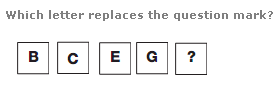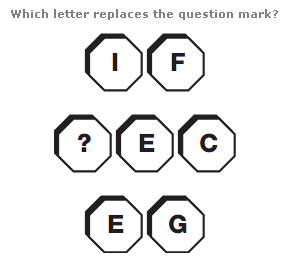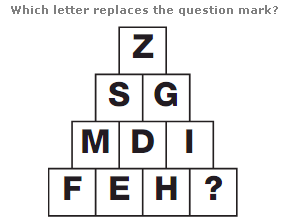# Puzzles - Missing letters puzzles

### Exercise :: Missing letters puzzlesAnswer : K Explanation : As you move down, the numerical value of the letters follows the sequence of Prime Numbers.Answer : A Explanation : Writing each letter as its numerical value and working in rows, add the top 2 digit number to the bottom 2 digit number to give the 3 digit result in the centre.Answer : G Explanation : The numerical values of the letters in each row add up to 26 each time.